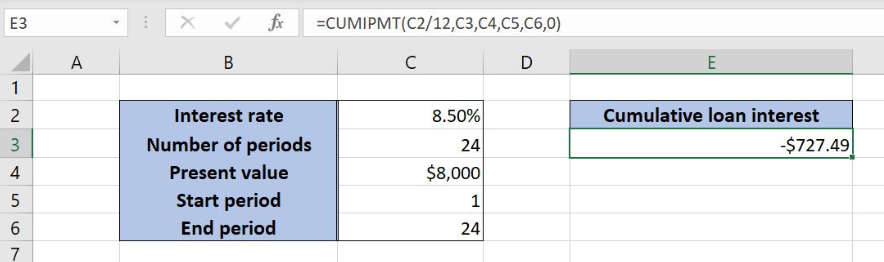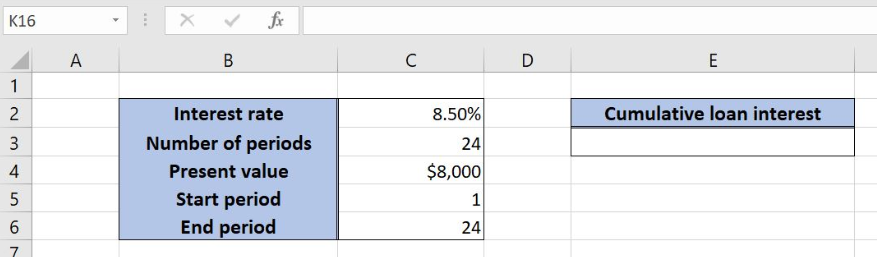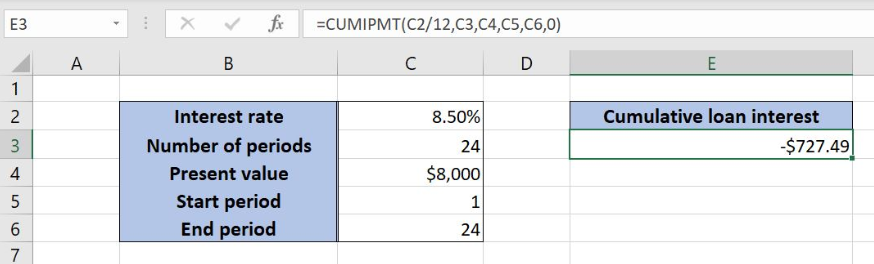Get instant live expert help with Excel or Google Sheets“My Excelchat expert helped me in less than 20 minutes, saving me what would have been 5 hours of work!”

#### Post your problem and you'll get expert help in seconds

Your message must be at least 40 characters
Our professional experts are available now. Your privacy is guaranteed.

# Calculate cumulative loan interest

Excel allows a user to calculate a cumulative loan interest, by using the CUMIPMT function. This step by step tutorial will assist all levels of Excel users in calculating a cumulative loan interest.Figure 1. The result of the CUMIPMT function

## Syntax of the CUMIPMT Formula

The generic formula for the CUMIPMT function is:

`=CUMIPMT(rate, nper, pv, start_period, end_period, type)`

The parameters of the CUMIPMT function are:

• rate – an interest rate for the period (the annual rate divided by 12)
• nper – the total number of payments for a loan
• pv – the present value of a loan
• start_period – a start period of a loan
• end_period – an end period of a loan
• type –  specifies when the payments are made: at the end of a period (0) or at the beginning of a period (1).

## Setting up Our Data for the CUMIPMT FunctionFigure 2. Data that we will use in the CUMIPMT example

Let’s look at the structure of the data we will use. In the cell C2, we have the annual interest rate for the loan. In the cell C3, is the total number of payments, while in the C5 is the present value of the loan. Cells C5 and C6 contain the start and end period. We want to get the result of the CUMIPMT function in the cell E3.

## Calculate the Cumulative Loan Interest Using the CUMIPMT

In our example, we want to get the cumulative loan interest for the whole period of the loan in the cell E3. Because of that the start period is 1 and the end is 24. The interest rate is 8.50% and the present value of the loan is \$8,000.

The formula looks like:

`=CUMIPMT(C2/12 ,C3, C4, C5, C6, 0)`

The parameter rate is C2/12, as we must pass the monthly interest rate to the function. The nper is the cell C4 (24), while the start_period is C5 (1) and the end_period is C6 (24). The pv is in the C5 (\$8,000). The type is 0, as the payments are being made at the end of the period.

To apply the CUMIPMT function, we need to follow these steps:

• Select cell E3 and click on it
• Insert the formula: `=CUMIPMT(C2/12 ,C3, C4, C5, C6, 0)`
• Press enterFigure 3. Using the CUMPIPMT function to calculate the cumulative loan interest

Finally, the result in the cell E3 is -727.49, which is the cumulative loan interest. The result is always negative, as it is the payment.

Most of the time, the problem you will need to solve will be more complex than a simple application of a formula or function. If you want to save hours of research and frustration, try our live Excelchat service! Our Excel Experts are available 24/7 to answer any Excel question you may have. We guarantee a connection within 30 seconds and a customized solution within 20 minutes.

### Did this post not answer your question? Get a solution from connecting with the expert.Another blog reader asked this question today on Excelchat:## Subscribe to Excelchat.coAnother blog reader asked this question today on Excelchat: